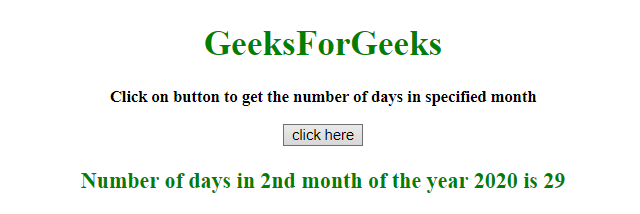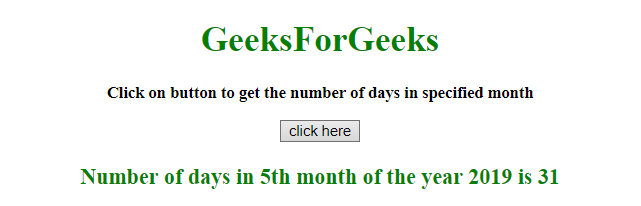# How to get the number of days in a specified month using JavaScript ?

Given a month and the task is to determine the number of days of that month using JavaScript.

• JavaScript getDate() Method: This method returns the number of days in a month (from 1 to 31) for the defined date.

Syntax:

`Date.getDate()`

Return value: It returns a number, from 1 to 31, representing the day of the month.

• JavaScript getFullYear() Method: This method returns the year (four digits for dates between year 1000 and 9999) of the defined date.

Syntax:

`Date.getFullYear()`

Return value: It returns a number, representing the year of the defined date

• JavaScript getMonth() Method: This method returns the month (from 0 to 11) for the defined date, based on to local time.

Syntax:

`Date.getMonth()`

Return value: It returns a number, from 0 to 11, representing the month.

Example 1: This example gets the days in the month (february) of the year (2020) by passing the month (1-12) and year to the function daysInMonth.

 `  ` `<``html``>  ` `    ``<``head``>  ` `        ``<``title``>  ` `            ``Get number of days in ` `            ``a specified month ` `        ``      ` `    ``  ` `     `  `    ``<``body` `style` `= ``"text-align:center;"``> ` `          `  `        ``<``h1` `style` `= ``"color:green;"` `>  ` `            ``GeeksForGeeks  ` `        `` ` `         `  `        ``<``p` `id` `= ``"GFG_UP"` `style` `= ` `            ``"font-size: 15px; font-weight: bold;"``> ` `        `` ` `         `  `        ``<``button` `onclick` `= ``"GFG_Fun()"``> ` `            ``click here ` `        `` ` `         `  `        ``<``p` `id` `= ``"GFG_DOWN"` `style` `= ` `            ``"color:green; font-size: 20px; font-weight: bold;"``> ` `        `` ` `         `  `        ``<``script``> ` `            ``var up = document.getElementById('GFG_UP'); ` `             `  `            ``up.innerHTML = "Click on button to get the" ` `                ``+ " number of days in specified month"; ` `                 `  `            ``var down = document.getElementById('GFG_DOWN');  ` `             `  `            ``function daysInMonth (month, year) { ` `                ``return new Date(year, month, 0).getDate(); ` `            ``} ` `             `  `            ``function GFG_Fun() { ` `                ``var date = new Date(); ` `                ``var month = 2; ` `                ``var year = 2020; ` `                ``down.innerHTML = "Number of days in " + month ` `                             ``+ "nd month of the year " + year ` `                             ``+" is "+ daysInMonth(month, year); ` `            ``} ` `        ``  ` `    ``  ` `                     `

Output:

• Before clicking on the button:• After clicking on the button:Example 2: This example gets the days in the current month of the current year by passing the month (1-12) and year to the function daysInMonth.

 `  ` `<``html``>  ` `    ``<``head``>  ` `        ``<``title``>  ` `            ``Get number of days in a specified month ` `        ``      ` `    ``  ` `     `  `    ``<``body` `style` `= ``"text-align:center;"``>  ` `     `  `        ``<``h1` `style` `= ``"color:green;"` `>  ` `            ``GeeksForGeeks  ` `        `` ` `         `  `        ``<``p` `id` `= ``"GFG_UP"` `style` `=  ` `            ``"font-size: 15px; font-weight: bold;"``> ` `        `` ` `         `  `        ``<``button` `onclick` `= ``"GFG_Fun()"``> ` `            ``click here ` `        `` ` `         `  `        ``<``p` `id` `= ``"GFG_DOWN"` `style` `=  ` `            ``"color:green; font-size: 20px; font-weight: bold;"``> ` `        `` ` `         `  `        ``<``script``> ` `            ``var up = document.getElementById('GFG_UP'); ` `             `  `            ``up.innerHTML = "Click on button to get the number" ` `                    ``+ " of days in specified month"; ` `             `  `            ``var down = document.getElementById('GFG_DOWN');  ` `             `  `            ``function daysInMonth (month, year) { ` `                ``return new Date(year, month, 0).getDate(); ` `            ``} ` `             `  `            ``function GFG_Fun() { ` `                ``var date = new Date(); ` `                ``var month = date.getMonth() + 1; ` `                ``var year = date.getFullYear(); ` `                ``down.innerHTML = "Number of days in " + month ` `                            ``+ "th month of the year " + year  ` `                            ``+" is "+ daysInMonth(month, year); ` `            ``} ` `        ``  ` `    ``  ` `                     `

Output:

• Before clicking on the button:• After clicking on the button:My Personal Notes arrow_drop_upCheck out this Author's contributed articles.

If you like GeeksforGeeks and would like to contribute, you can also write an article using contribute.geeksforgeeks.org or mail your article to contribute@geeksforgeeks.org. See your article appearing on the GeeksforGeeks main page and help other Geeks.

Please Improve this article if you find anything incorrect by clicking on the "Improve Article" button below.